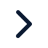Executive ProgramsWorkshopsProjectsBlogsCareersFind JobsCorporate TrainingHire from USAll Courses

Choose a category

All Courses

All Courses# Solving a second order ODE IVP in MATLAB and the simulation of a damped pendulum motion

MATLAB program to solve an 2nd order ODE Initial Value Problem and to simulate the motion of a pendulum for 20 seconds: 1. The YouTube link to the video demonstrating the animation of the damped oscillation of a simple pendulum in MATLAB is given below: https://youtu.be/-6JF_aQnPTI 2. (a) The syntax of the program is given…

Project Details

Thanks for choosing to leave a comment. Please keep in mind that all the comments are moderated as per our comment policy, and your email will not be published for privacy reasons. Please leave a personal & meaningful conversation.

Be the first to add a comment

Read more Projects by Priyotosh Bairagya (10)

Flow simulation of a 1D Super-Sonic nozzle using the Mac-Cormack method

Objective:

NUMERICAL SOLUTION OF 1D SUPERSONIC NOZZLE FLOW SIMULATION BY MAC-CORMACK METHOD PROJECT OBJECTIVES: i. Numerical solution of the governing equations in both conservative and non-conservative forms. ii. Creating user defined functions for calculating the flow quantities in both Conservative and Non-Conservative forms.…26 Nov 2019 03:36 PM IST

• MATLAB

Pipe flow simulation in OpenFOAM (Part 1/2: Wedge boundary conditions)

Objective:

LAMINAR INCOMPRESSIBLE FLOW SIMULATION THROUGH A PIPE IN OPENFOAM: [PART 1/2]: WEDGE BOUNDARY CONDITION: OBJECTIVES:1. Calculation of Pre-eliminary quantinites related to the flow.2. Creating the Mesh script (blockMeshDict file) for specifying the geometry and boundary condition.3. Simulation Results and post processing…10 Jan 2019 08:57 AM IST

Pipe flow simulation in OpenFOAM (Part 2/2: Symmetry boundary conditions)

Objective:

LAMINAR INCOMPRESSIBLE FLOW SIMULATION THROUGH A PIPE IN OPENFOAM: [PART 2/2]: SYMMETRY BOUNDARY CONDITION: OBJECTIVES:1. Creating the Mesh-Script (blockMeshDict file) with symmetry boundary condition.2. Simulation Results and post processing the velocity profile at different points of the pipe for fully developed flow.…08 Oct 2018 01:46 PM IST

Analysis of Solution Stability in a 2D Heat conduction problem

Objective:

ANALYSIS OF NUMERICAL STABILITY OF VARIOUS ITERATIVE SOLVERS FOR TRANSIENT 2D HEAT CONDUCTION: [Part: 3/3] INTRODUCTION: The criterion of stability of a numerical scheme is determined by the way the errors propagate while the solution moves from one time-step to the next in case of a transient solver. While solving governing…21 Sep 2018 04:55 AM IST

BlockMesh Analysis of a Backward Facing Step

Objective:

MESH GENERATION AND ANALYSIS USING BLOCKMESH FOR FLOW OVER A BACKWARD FACING STEP: The purpose of the following project is to generate the geometry for a variation of the incompressible cavity flow problem in OpenFOAM. For this purpose we have modified the lid-driven cavity tutorial in OpenFOAM to generate the mesh. We…21 Sep 2018 03:54 AM IST

Analysis of Steady and Unsteady State solutions of a 2D Heat conduction problem

Objective:

ANALYSIS OF VARIOUS ITERATIVE SCHEMES FOR THE SOLUTION OF A 2D HEAT CONDUCTION PROBLEM: [PART: 2/3] In the previous part we had explained the problem statement and the MATLAB Program in detail. In this Part we are going to explain the outputs from the 2D Heat Conduction program. A. Steady State Solutions: The Temperature…16 Sep 2018 08:27 AM IST

Objective:

Simulation of a 2D Heat Conduction problem in steady and unsteady/transient forms using iterative methods. Project Objectives: 1. Solving the 2 Dimensional Heat conduction equation in the generalized form using various iterative techniques: i. Explicit Solver (for unsteady state) ii. Implicit Solvers (for both steady and…16 Sep 2018 06:29 AM IST

Iterative solution of a system of linear equations and an analysis of spectral radius of a matrix

Objective:

UNDERSTANDING LINEAR SYSTEMS(ANALYSIS OF VARIOUS ITERATIVE SCHEMES TO SOLVE A SYSTEM OF LINEAR EQUATIONS TO FIND THE EIGEN VALUES AND SPECTRAL RADIUS) (A) PROBLEM STATEMENT: Given coefficient matrix: A = [[5,1,2],[-3,9,4],[1,2,-7]] Given Solution Matrix: X = [[x1],[x2],[x3]] Given RHS Matrix: B = [,[-14],]…31 Aug 2018 07:33 AM IST

Solving a second order ODE IVP in MATLAB and the simulation of a damped pendulum motion

Objective:

MATLAB program to solve an 2nd order ODE Initial Value Problem and to simulate the motion of a pendulum for 20 seconds: 1. The YouTube link to the video demonstrating the animation of the damped oscillation of a simple pendulum in MATLAB is given below: https://youtu.be/-6JF_aQnPTI 2. (a) The syntax of the program is given…30 Jul 2018 04:31 PM IST

Program in MATLAB to calculate the error in approximation of 1st order 2nd order and 4th order methods

Objective:

The following are the link to a MATLAB Program that calculates error in approximation. The error calculation happens in an user defined function: The google drive link to the MATLAB program that calculates the error in approximation of the first derivative using first order, second order and fourth order approximations…30 Jul 2018 04:05 PM IST

Showing 1 of 10 projects
Try our top engineering courses, projects & workshops today!